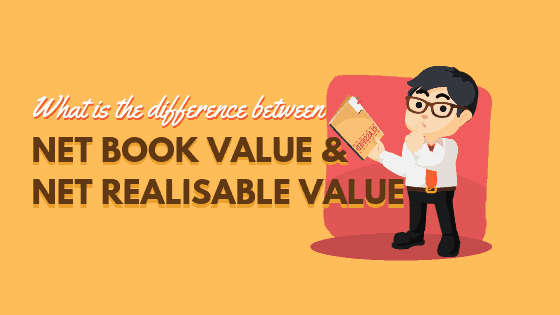# What is the difference between Net Book Value (NBV) and Net Realisable Value (NRV)?

The Net Book Value (NBV), also known as depreciated cost, is equal to its original cost (its book value) less amortisation (not in O’/N’ level syllabus) and depreciation. On the other hand, the Net Realisable Value (NRV) refers to the selling price of an asset minus the expenses incurred in the sales transaction, and in…

The Net Book Value (NBV), also known as depreciated cost, is equal to its original cost (its book value) less amortisation (not in O’/N’ level syllabus) and depreciation.

On the other hand, the Net Realisable Value (NRV) refers to the selling price of an asset minus the expenses incurred in the sales transaction, and in bringing the asset to the saleable state. NRV is most often used when the value of stock is less than the its historical cost.

Note on Net Realisable Value
In the examination where both the cost of purchase and the NRV (or market value) of the stock is available, use the figure that is lower. The fancy way of saying it is the ‘lower of cost or market value’ rule.
This follows the accounting principle of prudence and conservatism, where assets are not overstated and liabilities not understated.

Image Credit:

Inventory